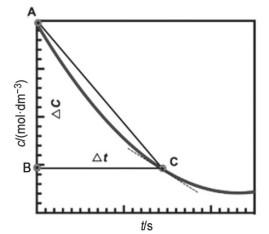## Discussion on Methodic Error of the Reaction between Acetone and Iodine

Han Li,, Jin Xin, Zhang Wei

 基金资助: 上海交通大学教学发展中心教学发展基金.  CTLD18B 0003

 Fund supported: 上海交通大学教学发展中心教学发展基金.  CTLD18B 0003Abstract

The methodic error of reaction between acetone and iodine was investigated using a simple and novel method. The relative error was calculated to as low as 10-4. We also give a discussion on the reason causing this error.

Keywords： Reaction between acetone and iodine ; Mean reaction rate ; Momentary reaction rate ; Relative error

Han Li. Discussion on Methodic Error of the Reaction between Acetone and Iodine. University Chemistry[J], 2020, 35(2): 43-45 doi:10.3866/PKU.DXHX201905048

$\text{C}{{\text{H}}_{\text{3}}}\text{COC}{{\text{H}}_{\text{3}}}\left( \text{aq} \right)+{{\text{I}}_{\text{2}}}\left( \text{aq} \right)\to \text{C}{{\text{H}}_{\text{3}}}\text{COC}{{\text{H}}_{\text{2}}}\text{I}\left( \text{aq} \right)+{{\text{H}}^{\text{+}}}\left( \text{aq} \right)+{{\text{I}}^{-}}\left( \text{aq} \right)$

### 图1$\left| \bar{v}-{{v}_{0}} \right|\le \left| {{v}_{t}}-{{v}_{0}} \right|$

(1)碘开始反应时(图1中0时刻)，此时丙酮初始浓度为${{c}_{0\left(\text{acetone} \right)}}$，反应速率为v0，溶液体系中丙酮初始量为：

4.00 × 10.0 × 10−3 = 4.00 × 10−2 (mol)

(2)碘反应完(图1t时刻)，此时丙酮浓度为${{c}_{t\left(\text{acetone} \right)}}$，反应速率为vt，根据式(1)中的计量关系，碘全部反应完成消耗的丙酮量为：

0.00118 × 15.0 × 10−3 = 1.77 × 10−5 (mol)

(3)误差大小估算。

vtv0之间的相对误差为：

 编号 V丙酮/cm3 V盐酸/cm3 V碘 /cm3 V水/cm3 v0/(mol·dm−3·s−1) vt/(mol·dm−3·s−1) $\bar{v}$与v0相对误差的绝对值 1 10.0 10.0 15.0 15.0 0.800k 0.7996k ≤ 0.0443% 2 10.0 10.0 10.0 20.0 0.800k 0.7998k ≤ 0.0280% 3 5.0 10.0 15.0 20.0 0.400k 0.3996k ≤ 0.0885%

## 参考文献 原文顺序 文献年度倒序 文中引用次数倒序 被引期刊影响因子

/

 〈〉